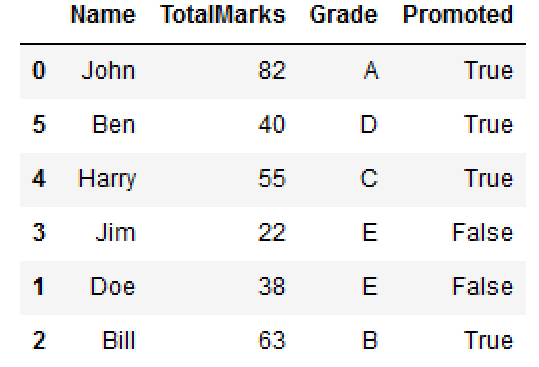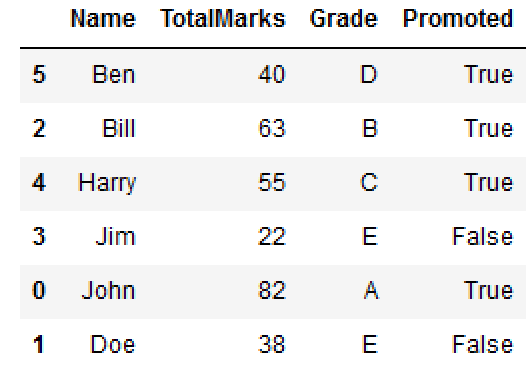Net-informations.com

# Randomly Shuffle DataFrame Rows in Pandas

You can use the following methods to shuffle DataFrame rows:

1. Using pandas
1. Using numpy
1. Using sklearn

Lets create a DataFrame..

## pandas.DataFrame.sample()

Shuffling the rows of the Pandas DataFrame using the sample() method with the parameter frac, The frac argument specifies the fraction of rows to return in the random sample.Argument frac=1 means return all rows.

If you wish to shuffle your dataframe in-place and reset the index, you could do e.g.

## numpy.random.permutation()

Here, specifying drop=True prevents .reset_index from creating a column containing the old index entries.

## sklearn.utils.shuffle()net-informations.com (C) 2022    Founded by raps mk